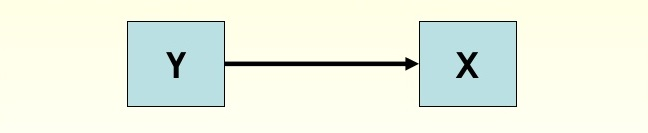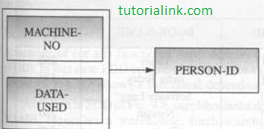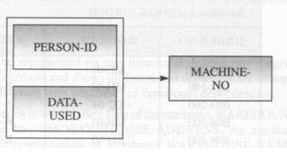DBMS

# Functional Dependency Diagram and Examples

In a functional dependency diagram (FDD), functional dependency is represented by rectangles  representing attributes and a heavy arrow showing dependency. Fig. shows A functional dependency diagram for the simplest functional dependency, that is, FD: Y  ->  X.
In functional dependency diagram, each FD is displayed as a horizontal line.
The left-hand side attributes  of the FD, i.e. determinants, are connected by Vertical lines to line representing the FD.
The right-hand side attributes are connected by arrows pointing towards the attibutes.Relation schema R along with FD: Y -> X

### Example:

```Let us consider a functional dependency that there is one person working on a machine  each day, which is given as:
FD: {MACHINE-NO,DATA-USED} -> {PERSON-ID}
It means that once the values of MACHINE-NO and DATA-USED are known, a unique value
of PERSON-ID also can be known. Fig.(a) shows the functional dependency diagram (FDD)
for this example.
```(a)(b)

Similarly, in the above example, if the person also uses one machine each day, then FD can be given as:
FD: {PERSON-ID, DATA-USED} -> {MACHINE-NO}
It means that once the values of PERSON-ID and DATA-USED are known, a unique value of MACHINE-NO also can be known. Fig. (b) shows the functional dependency diagram (FDD) for this example